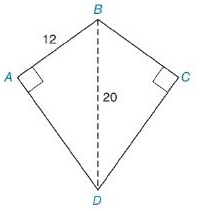Chapter 8.2, Problem 18EElementary Geometry For College St...

7th Edition
Alexander + 2 others
ISBN: 9781337614085

Solutions

Chapter
SectionElementary Geometry For College St...

7th Edition
Alexander + 2 others
ISBN: 9781337614085
Textbook Problem

In Exercises 13 to 18, find the area of the given polygon.Kite A B C D

To determine

To Find:

Area of the kite.

Explanation

Formula Used:

Pythagorean theorem for the right angle triangle ABC for the hypotenuse AC,

AC2=AB2+BC2.

Calculation:

It is given that the kite ABCD has one of the diagonals BD=d1=20 and the side AB=12 with mBAD=90°,mBCD=90°.

Here the kite is symmetric with respect to the longest diagonal.

Still sussing out bartleby?

Check out a sample textbook solution.

See a sample solution

The Solution to Your Study Problems

Bartleby provides explanations to thousands of textbook problems written by our experts, many with advanced degrees!

Get Started

Let f(x) = {x21if2x1x3if1x2 Find (a) limx1 f(x) and limx1+ f(x). Is f continuous at x = 1? Explain.

Applied Calculus for the Managerial, Life, and Social Sciences: A Brief Approach

If f is continuous on [0, 1], prove that 01f(x)dx=01f(1x)dx

Single Variable Calculus: Early Transcendentals, Volume I

(f(x)g(x)) = f(x)g(x).

Study Guide for Stewart's Single Variable Calculus: Early Transcendentals, 8th